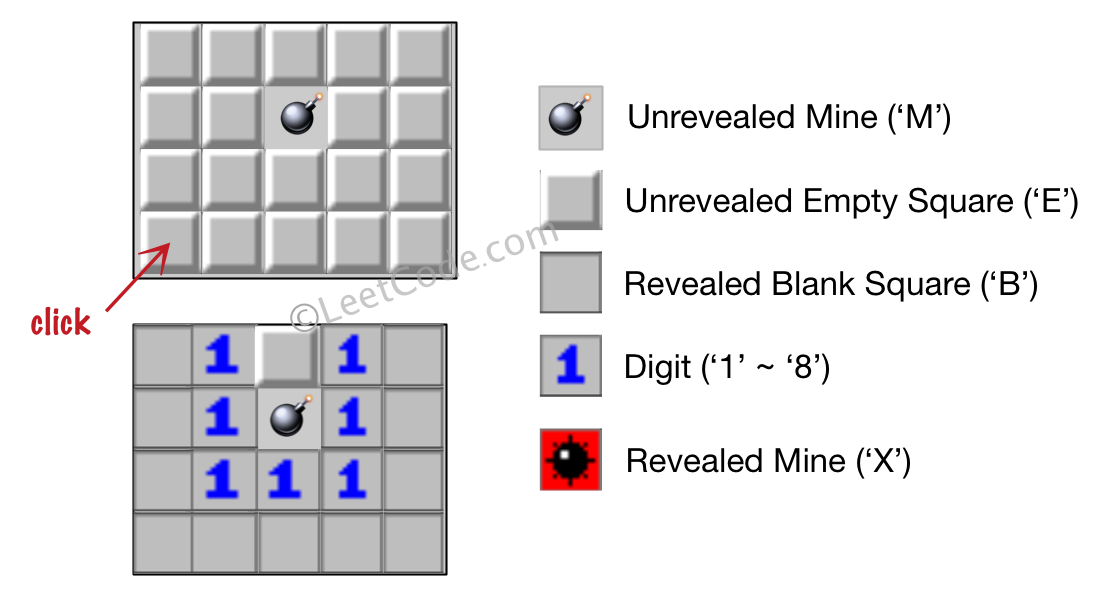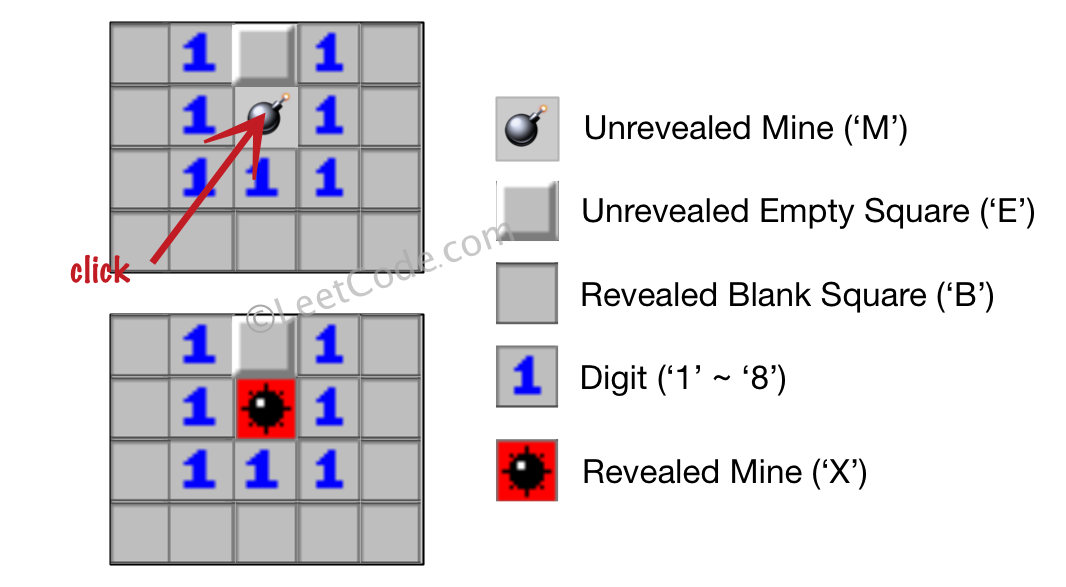LeetCode: 529. 扫雷游戏¶

1、题目描述¶

[['E', 'E', 'E', 'E', 'E'],
['E', 'E', 'M', 'E', 'E'],
['E', 'E', 'E', 'E', 'E'],
['E', 'E', 'E', 'E', 'E']]

Click : [3,0]

[['B', '1', 'E', '1', 'B'],
['B', '1', 'M', '1', 'B'],
['B', '1', '1', '1', 'B'],
['B', 'B', 'B', 'B', 'B']][['B', '1', 'E', '1', 'B'],
['B', '1', 'M', '1', 'B'],
['B', '1', '1', '1', 'B'],
['B', 'B', 'B', 'B', 'B']]

Click : [1,2]

[['B', '1', 'E', '1', 'B'],
['B', '1', 'X', '1', 'B'],
['B', '1', '1', '1', 'B'],
['B', 'B', 'B', 'B', 'B']]• 输入矩阵的宽和高的范围为 [1,50]。

• 点击的位置只能是未被挖出的方块 ('M' 或者 'E')，这也意味着面板至少包含一个可点击的方块。

• 输入面板不会是游戏结束的状态（即有地雷已被挖出）。

• 简单起见，未提及的规则在这个问题中可被忽略。例如，当游戏结束时你不需要挖出所有地雷，考虑所有你可能赢得游戏或标记方块的情况。

2、解题思路¶

• 使用DFS
• 判断当前坐标是否满足条件或者为E，否则跳出
• 统计当前坐标周围有多少个炸弹，如果有，更新数量
• 没有炸弹，则将该位置标记为B，并且递归周围的8个位置
class Solution:
def updateBoard(self, board: List[List[str]], click: List[int]) -> List[List[str]]:

x, y = click
surround = [(-1, -1), (-1, 0), (-1, 1), (0, -1), (0, 1), (1, -1), (1, 0), (1, 1)]

row = len(board)
col = len(board)

def available_pos(m, n):
return 0 <= m < row and 0 <= n < col

def dfs(a, b):
if not available_pos(a, b) or board[a][b] != "E":
return

bombs = 0

for da, db in surround:
if available_pos(a + da, b + db) and board[a + da][b + db] == "M":
bombs += 1
if bombs:
board[a][b] = str(bombs)
else:
board[a][b] = "B"
for da, db in surround:
dfs(a + da, b + db)

if board[x][y] == "M":
board[x][y] = "X"
else:
dfs(x, y)

return board